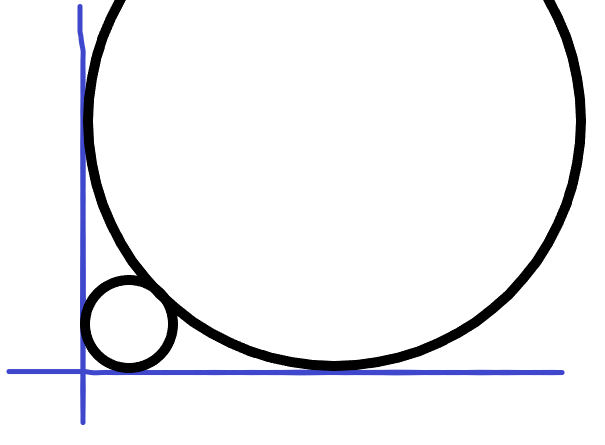# 圆——高精无理数的处理

• 在第一象限中依次画圆
• 第一个圆半径为 𝑅 且和两坐标轴相切
• 接下来每一个圆和前一个圆以及两个坐标轴相切
• 求第 𝑛 个圆的半径（保留整数部分末尾1000位）

• 𝑛 ≤ 10^18, 𝑅 ≤ 10^9$N=(3+2\sqrt{2})^n+(3-2\sqrt{2})^n$

$t=(3-2\sqrt{2})^n$

t本身是小于1的，

1.有序数对(a,b)的做法

2.$N=(3+2\sqrt{2})^n+(3-2\sqrt{2})^n$的转化，利用$(3-2\sqrt{2})<1$，从而使得要么变成0，要么在double可以承受的范围下。

posted @ 2018-10-06 21:23  *Miracle*  阅读(338)  评论(0编辑  收藏  举报